Just like the mixer module, the drawing API is fairly straightforward with a few examples. Therefore instead of re-iterating the documentation as part of this tutorial, I'll instead show you a few simple (and not-so-simple) examples of what can be doing with the draw module in PyGame and a few pitfalls to be aware of. At the end of this tutorial/demo you'll see a massive code dump of a sample application. Simply run this script and you'll be presented with a PyGame app that is a sequence of draw module demos.

While running it, press spacebar to proceed through the demos.

## Demo 1: Rectangle

pygame.draw.rect(surface, color, pygame.Rect(left, top, width, height))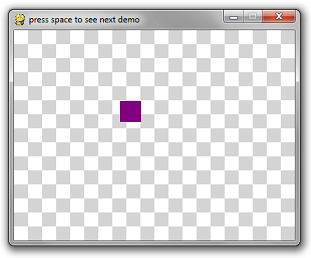## Demo 2: Circle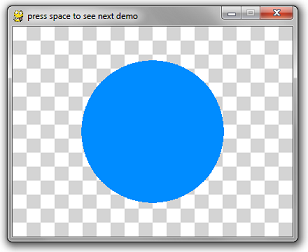## Demo 3: Built in Outlines:

This is the first caveat you should be aware of. PyGame's method for creating "thicker" outlines for circles is to draw multiple 1-pixel outlines. In theory, it sounds okay, until you see the result:The circle has noticeable pixel gaps in it. Even more embarrassing is the rectangle, which uses 4 line-draw calls at the desired thickness. This creates weird corners.

The way to do this for most drawing API calls is to pass in an optional last parameter which is the thickness.
# draw a rectangle
pygame.draw.rect(surface, color, pygame.Rect(1010100100), 10)
# draw a circle
pygame.draw.circle(surface, color, (30060), 5010)

Moral of the story: when you draw a polygon, rectangle, circle, etc, draw it filled in or with 1-pixel thickness. Everything else is not very well implemented.

## Demo 4: Acceptable Outlines

If you must draw a rectangle that has 10-pixel-thick borders, then it's best that you re-implement the logic yourself with either 10 1-pixel-thick rectangle calls, or 4 10-pixel-thick rectangle calls for each side.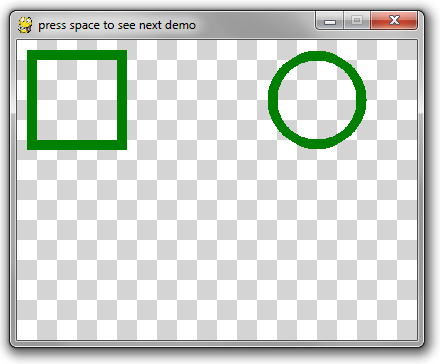For an example, see the do_nice_outlines function below.

## Demo 5: Polygons

This API is pretty straightforward. The point list is a list of tuples of x-y coordinates for the polygon.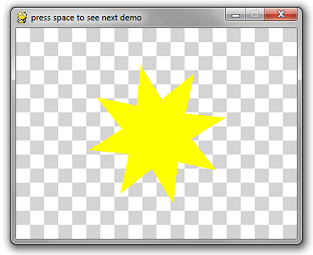pygame.draw.polygon(surface, color, point_list)

## Demo 6: Lines

Lines are also straight-forward:
pygame.draw.line(surface, color, (startX, startY), (endX, endY), width)

So I decided to go a bit crazy and wrote a 3D spinning wireframe cube using the line method and a lot of math.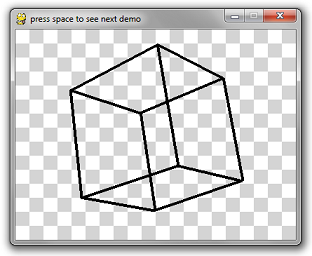import pygame
import math
import time

# Ignore these 3 functions. Scroll down for the relevant code.

def create_background(width, height):
colors = [(255255255), (212212212)]
background = pygame.Surface((width, height))
tile_width = 20
y = 0
while y < height:
x = 0
while x < width:
row = y // tile_width
col = x // tile_width
pygame.draw.rect(
background,
colors[(row + col) % 2],
pygame.Rect(x, y, tile_width, tile_width))
x += tile_width
y += tile_width
return background

def is_trying_to_quit(event):
pressed_keys = pygame.key.get_pressed()
alt_pressed = pressed_keys[pygame.K_LALT] or pressed_keys[pygame.K_RALT]
x_button = event.type == pygame.QUIT
altF4 = alt_pressed and event.type == pygame.KEYDOWN and event.key == pygame.K_F4
escape = event.type == pygame.KEYDOWN and event.key == pygame.K_ESCAPE
return x_button or altF4 or escape

def run_demos(width, height, fps):
pygame.init()
screen = pygame.display.set_mode((width, height))
pygame.display.set_caption('press space to see next demo')
background = create_background(width, height)
clock = pygame.time.Clock()
demos = [
do_rectangle_demo,
do_circle_demo,
do_horrible_outlines,
do_nice_outlines,
do_polygon_demo,
do_line_demo
]
the_world_is_a_happy_place = 0
while True:
the_world_is_a_happy_place += 1
for event in pygame.event.get():
if is_trying_to_quit(event):
return
if event.type == pygame.KEYDOWN and event.key == pygame.K_SPACE:
demos = demos[1:]
screen.blit(background, (00))
if len(demos) == 0:
return
demos(screen, the_world_is_a_happy_place)
pygame.display.flip()
clock.tick(fps)

# Everything above this line is irrelevant to this tutorial.

def do_rectangle_demo(surface, counter):
left = (counter // 2) % surface.get_width()
top = (counter // 3) % surface.get_height()
width = 30
height = 30
color = (1280128# purple

# Draw a rectangle
pygame.draw.rect(surface, color, pygame.Rect(left, top, width, height))

def do_circle_demo(surface, counter):
x = surface.get_width() // 2
y = surface.get_height() // 2
max_radius = min(x, y) * 4 // 5
radius = abs(int(math.sin(counter * 3.14159 * 2 / 200) * max_radius)) + 1
color = (0140255# aquamarine

# Draw a circle

def do_horrible_outlines(surface, counter):
color = (25500# red

# draw a rectangle
pygame.draw.rect(surface, color, pygame.Rect(1010100100), 10)

# draw a circle
pygame.draw.circle(surface, color, (30060), 5010)

def do_nice_outlines(surface, counter):
color = (01280# green

# draw a rectangle
pygame.draw.rect(surface, color, pygame.Rect(101010010))
pygame.draw.rect(surface, color, pygame.Rect(101010100))
pygame.draw.rect(surface, color, pygame.Rect(1001010100))
pygame.draw.rect(surface, color, pygame.Rect(1010010010))

# draw a circle
center_x = 300
center_y = 60
iterations = 150
for i in range(iterations):
ang = i * 3.14159 * 2 / iterations
x = center_x + dx
y = center_y + dy
pygame.draw.circle(surface, color, (x, y), 5)

def do_polygon_demo(surface, counter):
color = (2552550# yellow

num_points = 8
point_list = []
center_x = surface.get_width() // 2
center_y = surface.get_height() // 2
for i in range(num_points * 2):
if i % 2 == 0:
ang = i * 3.14159 / num_points + counter * 3.14159 / 60
x = center_x + int(math.cos(ang) * radius)
y = center_y + int(math.sin(ang) * radius)
point_list.append((x, y))
pygame.draw.polygon(surface, color, point_list)

def rotate_3d_points(points, angle_x, angle_y, angle_z):
new_points = []
for point in points:
x = point
y = point
z = point
new_y = y * math.cos(angle_x) - z * math.sin(angle_x)
new_z = y * math.sin(angle_x) + z * math.cos(angle_x)
y = new_y
# isn't math fun, kids?
z = new_z
new_x = x * math.cos(angle_y) - z * math.sin(angle_y)
new_z = x * math.sin(angle_y) + z * math.cos(angle_y)
x = new_x
z = new_z
new_x = x * math.cos(angle_z) - y * math.sin(angle_z)
new_y = x * math.sin(angle_z) + y * math.cos(angle_z)
x = new_x
y = new_y
new_points.append([x, y, z])
return new_points

def do_line_demo(surface, counter):
color = (000# black
cube_points = [
[-1, -11],
[-111],
,
[1, -11],
[-1, -1, -1],
[-11, -1],
[11, -1],
[1, -1, -1]]

connections = [
(01),
(12),
(23),
(30),
(45),
(56),
(67),
(74),
(04),
(15),
(26),
(37)
]

t = counter * 2 * 3.14159 / 60 # this angle is 1 rotation per second

# rotate about x axis every 2 seconds
# rotate about y axis every 4 seconds
# rotate about z axis every 6 seconds
points = rotate_3d_points(cube_points, t / 2, t / 4, t / 6)
flattened_points = []
for point in points:
flattened_points.append(
(point * (1 + 1.0 / (point + 3)),
point * (1 + 1.0 / (point + 3))))

for con in connections:
p1 = flattened_points[con]
p2 = flattened_points[con]
x1 = p1 * 60 + 200
y1 = p1 * 60 + 150
x2 = p2 * 60 + 200
y2 = p2 * 60 + 150

# This is the only line that really matters
pygame.draw.line(surface, color, (x1, y1), (x2, y2), 4)

run_demos(40030060)

Next up: Fonts and Text

Hey, there, Python folks. Hope you enjoyed the post. I just wanted to give a quick shout-out for a weekly Python code golf that I recently started up over on StringLabs.io. If you like Python puzzles please come check it out!